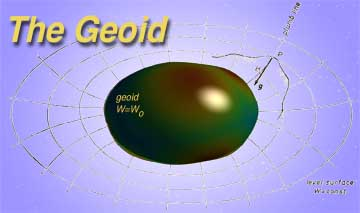Definitions

In Bomford's text, Geodesy (p.94), we find a definition of the concept of the geoid:

The geoid may be described as a surface coinciding with mean sea-level in oceans, and lying under the land at the level to which the sea would reach if admitted by small frictionless channels. More precisely, it is that equipotential surface of the Earth's attraction and rotation which, on average, coincides with mean sea-level in the open ocean.

Vanicek & Krakiwsky, in their Geodesy, The Concepts (p. 87), offers the following:

The one gravity equipotential surface of particular interest is that which best approximates the (mean) sea level over the whole Earth. It is called the geoid. Gauss described the geoid as being the mathematical figure of the Earth, and as such it is a key surface in geodesy, playing a fundamental role in positioning.

In Torge's book, Geodesy (p. 44), we find a similar description of the geoid:

We consider the waters of the ocean as freely moving homogeneous matter, which is subject only to the force of gravity of the Earth. Upon attaining a state of equilibrium, the surface of such idealized oceans assumes a level surface of the gravity field; we may regard it as being extended under the continents (e.g., by a system of communicating tubes). This level surface is termed the geoid.

Finally, Torge's more recent book, Gravimetry (p. 32), we find a succinct definition:

The level surface which optimally approximates mean sea level is denoted as geoid. It serves as a reference surface for defining height systems.

Maps

Last updated: November 12, 1999

Hosted by www.Geocities.ws